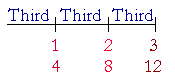S k i l l
i n
A R I T H M E T I C

Lesson 17  Section 2

# PROPORTIONS

 3. What is a proportion? A statement that two ratios are the same.

Example 1.   5 is to 15  as  8 is to 24.

That is a proportion because 5 is the third part of 15, just as 8 is the third part of 24.  (Lesson 15.)

A proportion involves four numbers called the terms.

1st is to 2nd  as  3rd is to 4th.

To explain why they are proportional we start with the first term and state its ratio to the second; we then state that the third term has that same ratio to the fourth.

Example 2.   Why is this a proportion?

16 is to 2  as  80 is to 10.

Answer.  This is a proportion because 16 is eight times 2, just as 80 is eight times 10.

Example 3.   Why is this a proportion?

10 is to 15  as  2 is to 3.Answer.  This is a proportion because 10 is two thirds of 15, just as 2 is two thirds of 3. (Lesson 15.)

Example 4.   Complete this proportion:

8 is to 32  as  9 is to ?

Solution.   8 is the fourth part of 32.  And 9 is the fourth part of 36.

The 1st term is to the 2nd  as  the 3rd is to the 4th.

Example 5.   Complete this proportion:

27 is to 3  as  ? is to 5

Solution.   27 is nine times 3.  And 45 is nine times 5.

If the student will speak and use sentences, the answer will be clear.

Example 6.   In each item below, what ratio has a to b?

(a simply means the first term; b means the second.)

a)  a is to b  as  1 is to 6.

b)  a is to b  as  10 is to 1.

a)  Since 1 is the sixth part of 6, then a is the sixth part of b.

b)  Since 10 is ten times 1, then a is ten times b.

Symbolic representation

The following is the traditional way to symbolize a proportion:

1 : 2 = 4 : 8.

"1 is to 2 as 4 is to 8."

Why is this a proportion?  Because 1 is half of 2, and 4 is half of 8.

Again, a proportion has 4 numbers -- 4 terms. The 1st, the 2nd, the 3rd, the 4th. In any problem, we are typically asked to find the 4th, which is called the 4th proportional.

Example 7.   Read this proportion, and complete it:

8 : 2 = 20 : ?

Answer.  "8 is to 2 as 20 is to what number?"

Now, what ratio has 8 to 2?

8 is four times 2.  And 20 is four times 5.

We say that we have solved that proportion. That is, given three terms, we have named the fourth.

Example 8.   Read this proportion and complete it:

7 : 21 = 4 : ?

Answer.  "7 is to 21 as 4 is to what number?"

What ratio has 7 to 21?

7 is the third part of 21.  And 4 is the third part of 12.

Example 9.   Complete this proportion:

2 : 3 = ? : 12

Answer.  "2 is to 3 as what number is to 12?"

Now, 2 is two thirds of 3.  What number is two thirds of 12?"One third of 12 is 4. Therefore, two thirds are 8.

2 : 3 = 8 : 12.

In the next lesson we will see how to solve a proportion that looks like this:

5 : 7 = 20 : ?

At this point, please "turn" the page and do some Problems.

or

Continue on to the next Section.

Previous Section

1st Lesson on Parts of Natural Numbers